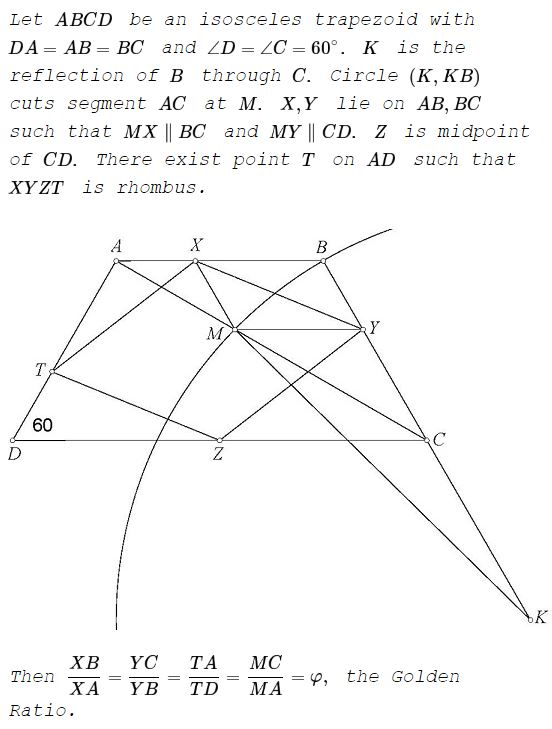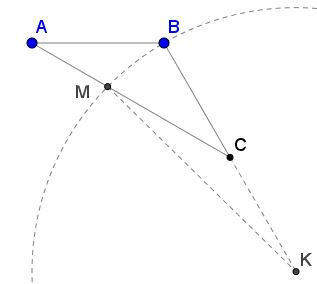# Golden Ratio in an Isosceles Trapezoid with a 60° Angle

### Source### Proof 1

WLOG, assume $A=-1+i\sqrt{3},\;$ $B=1+i\sqrt{3},\;$ $C=-2,\;$ $D=4.\;$ THis gives $K=3-i\sqrt{3}.\;$ The circle $(K, BK\;$ is given by $(x-3)^2+(y+\sqrt{3})^2=16.\;$ But $AC\;$ is described by $x=2-y\sqrt{3}.\;$ Let's find their intersections. On $AC,\;$ the circle's equation reduces to $(1+y\sqrt{3})^2+(y+\sqrt{3})^2=16,\;$ leading to a quadratic equation: $y^2+y\sqrt{3}-3,\;$ with the only positive root $\displaystyle y_M=\sqrt{3}\frac{-1+\sqrt{5}}{2}=\frac{\sqrt{3}}{\varphi}.\;$

For now, we are not interested in $x\;$ values. Clearly, $\displaystyle y_Y=y_M=\frac{\sqrt{3}}{\varphi}.\;$ By Thales' theorem, $\displaystyle\frac{MC}{MA}=\frac{YC}{YA}=\varphi.\;$ Now, $\displaystyle X_M=2-\frac{3}{\varphi}=5-3\varphi.\;$ Since $BC\;$ is described by $x\sqrt{3}+y=2\sqrt{3},\;$ we find $x_Y=3-\varphi.$

Finally, as $XBYM\;$ is a parallelogram, $x_X=3-2\varphi.\;$ From here, $\displaystyle\frac{XB}{XA}=\varphi.\;$ But $Z=0,\;$ implying $\displaystyle y_T=\sqrt{3}\left(1-\frac{1}{\varphi}\right)=\frac{\sqrt{3}}{\varphi^2}.\;$ Thus $\displaystyle\frac{TA}{TB}=\varphi.$

### Reformulation

As the matter of existence of point $T\;$ and the rhombus has been dealt with elsewhere, it is obvious that the only ratio that has to be verified is $\displaystyle\frac{MC}{MA}.\;$

The diagram and the statement could be simplified:

In $\Delta ABK,\;$ $AB=1,\;$ $BK=2,\;$ $\angle ABK=120^{\circ}.\;$ Let $C\;$ be the midpoint of $BK.\;$ $M\;$ a point on $AC\;$ such that $KM=2.$Then $\displaystyle\frac{CM}{AM}=\varphi,\;$ the Golden Ratio.

### Proof 2

Indeed, $\angle KCM=150^{\circ}.\;$ Set $CM=x.\;$ In $\Delta CKM,\;$ according to the Law of Cosines,

$2^2=1^2+x^2-2x\cos 150^{\circ}=x^2+x\sqrt{3}+1.$

This gives a quadratic equation $x^2+x\sqrt{3}-3=0,\;$ with the only positive root $x=\displaystyle\frac{\sqrt{15}-\sqrt{3}}{2}=\sqrt{3}\frac{1}{\varphi}.$

But, applying the Law of Cosines in $\Delta ABC,\;$ we obtain $AC=\sqrt{3}.\;$ Such that, finally, $\displaystyle\frac{AC}{CM}=\frac{CM}{AM}=\varphi.$

### Acknowledgment

The problem above has been kindly posted by Tran Quang Hung at the CutTheKnotMath facebook page, followed by a proof (Proof 1) by Leo Giugiuc.### Golden Ratio